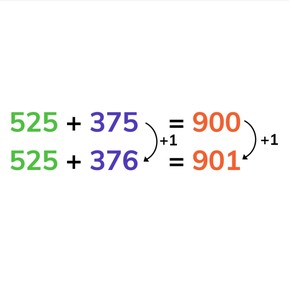Addition to 1,000 with one more or one less

# Addition to 1,000 with one more or one less8,000 schools use Gynzy92,000 teachers use Gynzy1,600,000 students use Gynzy

## General

Students learn to add to 1,000 using a strategy in which they either count one more or take one away.

2.NBT.B.7

## Relevance

Discuss with students that it is useful if you can add using one more or one less. If you know when to add one more, or take one away, you don't need to calculate sets of addition problems separately.

## Introduction

Show a set of number sequences on the board. The students must race against the timer to fill in all the missing numbers. Then students must determine what the neighbor numbers of the given numbers are.

## Development

Check that students are able to add to 1,000 with one more or one less by asking the following questions/exercises:
- What do you need to look closely at to know if you add one or take one away?
- Calculate the following addition problems: 28+600=, 28+599=
- Calculate the following addition problems: 715+185=, 714=185=,713+185=

## Guided practice

Students first practice adding with one more or one less with a set of two addition problems. Then students are asked to add one more or one less with a set of three addition problems.

## Closing

Discuss with students that it is important to be able to add to 1,000 with adding one more or one less, so that you can quickly solve a set of addition problems without solving each one separately. Show the addition problems on the interactive whiteboard and ask students to calculate. Ask how they solved the problems. Erase the grey boxes to reveal the answers.

## Teaching tips

Students who have difficulty with adding with one more or less can make use of MAB blocks to make it more visual. Ask students to set out the amount of blocks representing an addition problem. Then give them an addition problem that is one more. Show them that they simply add one block to their existing problem shown in blocks. Repeat this with one less.

## Instruction materials

MAB-blocks

### The online teaching platform for interactive whiteboards and displays in schools

• Save time building lessons

• Manage the classroom more efficiently

• Increase student engagement